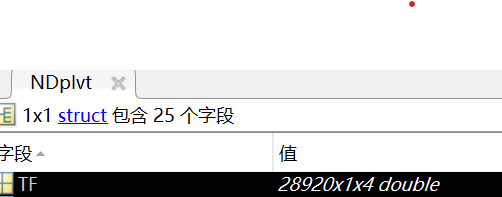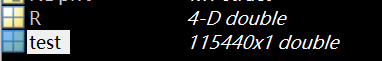# Failed to compress the connectivity matrix

hello,

I want to encode the connectivity matrix and save the connectivity matrix back in the TF variable following the tutorial. But I find the 'process_compress_sym' function don't compress the matrix correctly. The dimension and number of the variable is not compressed correctly. Can you please give me some instructions about this problem?
my test code is as below:

R = bst_memory('GetConnectMatrix', NDplvt);
test = process_compress_sym('Compress',R( : ) );

'test' is not equal to the initial 'NDplvt.TF' data.

Thank you very much.This procedure is described here:
https://neuroimage.usc.edu/brainstorm/Tutorials/Connectivity#On_the_hard_drive

If you think there is a problem with this function, please:

• Share the timefreq_connect_...m file with which you are having a problem (upload it somewhere and post the download link).
• Post a Matlab script that illustrates your concern (full code, from the file structure that you shared)

Thanks

Hello Francois,

Thanks for your help.

I try to use the tutorial code 'R = bst_memory('GetConnectMatrix', TfMat)',but i find the returned variable 'R' is a [Na x Nb x Ntime x 1] matrix instead of [Na x Nb x Ntime x Nfreqs (4 freqs in current TfMat)] one. The TfMat is uploaded.

Thanks!

timefreq_connectn_plv_220503_1529.mat (922.1 KB)

Continuing the discussion from Failed to compress the connectivity matrix:

hello Francois,

I have solved these problem, thanks!Fang

I downloaded your file, loaded it as `TfMat`.

``````>> R = bst_memory('GetConnectMatrix', TfMat);
>> size(R)
ans =
238   238     1     4
``````

Yes, I made a mistake here.

thank you for your help!

Fang